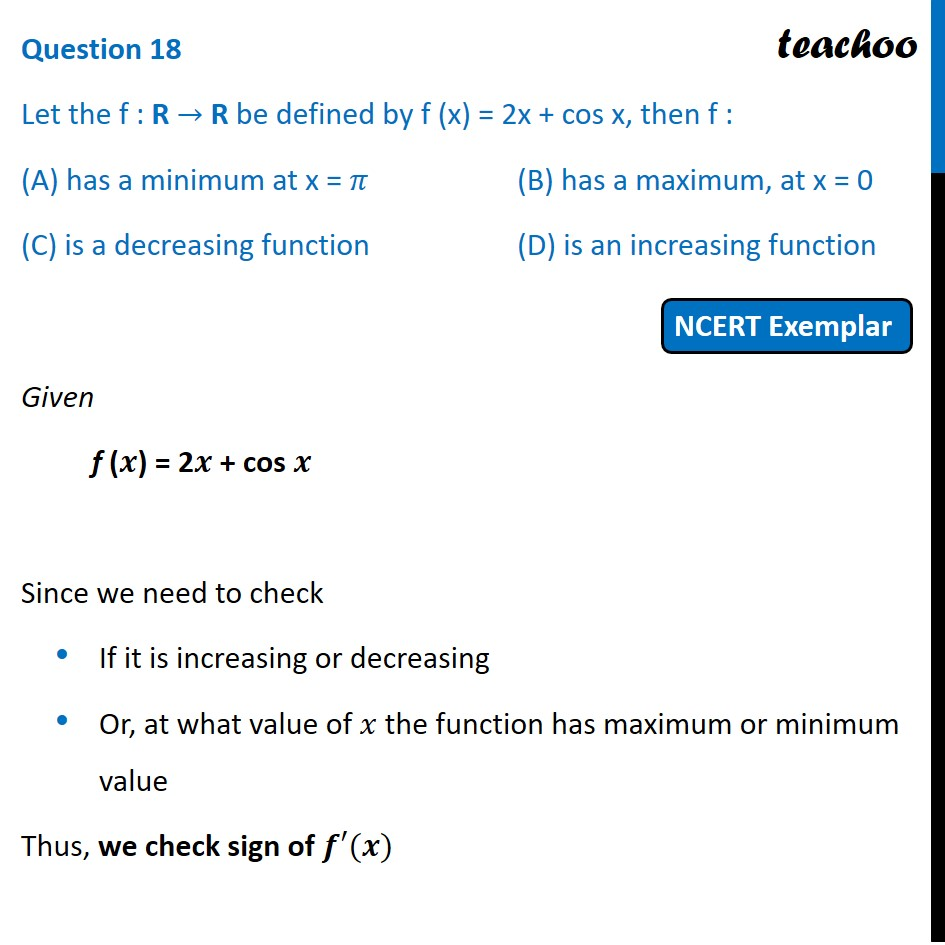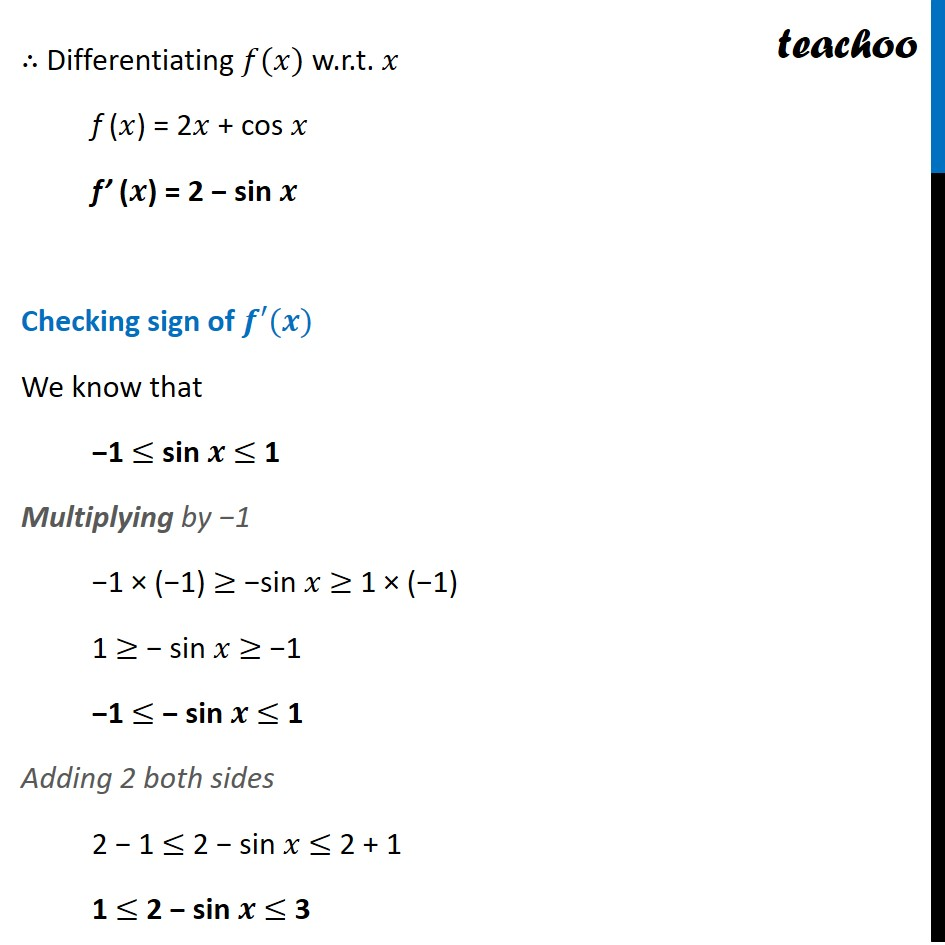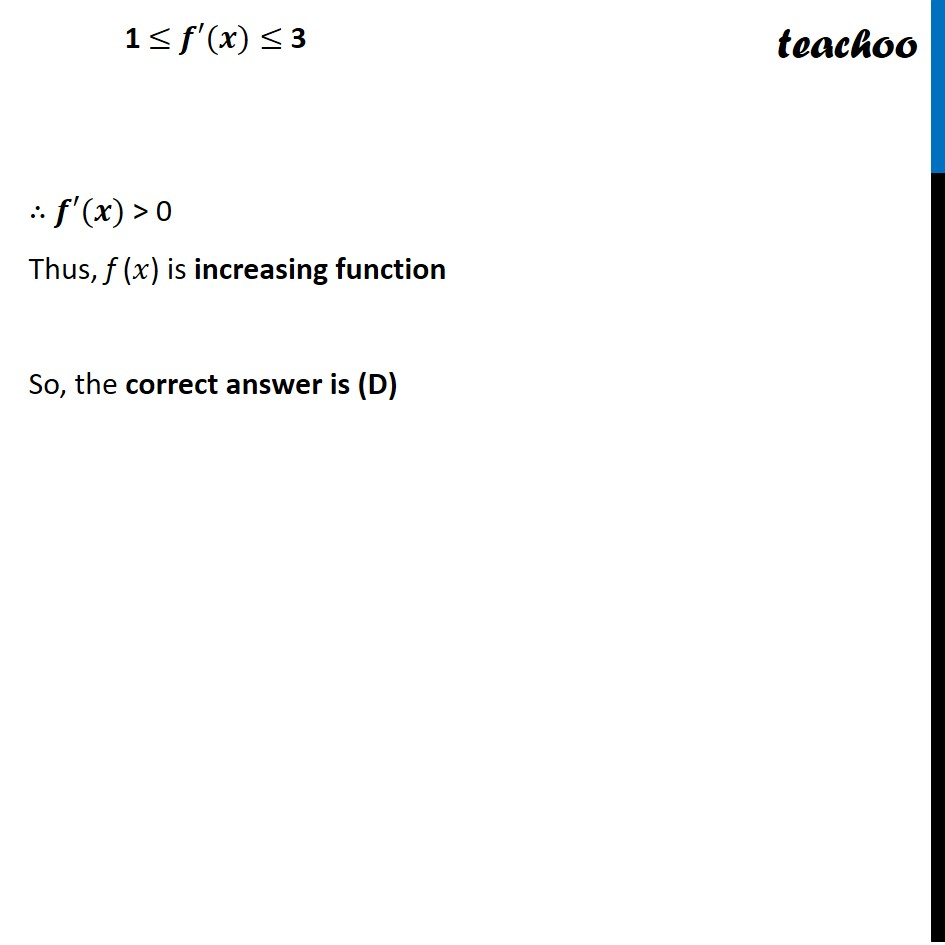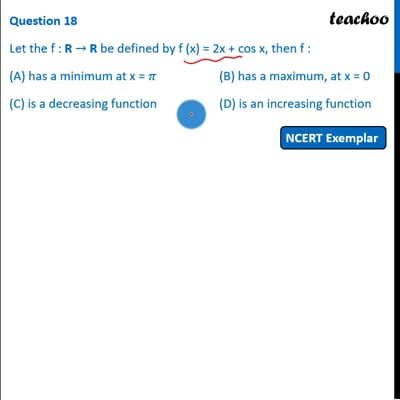NCERT Exemplar - MCQs

Chapter 6 Class 12 Application of Derivatives
Serial order wise

## (C) is a decreasing function         (D) is an increasing functionThis video is only available for Teachoo black users

Get live Maths 1-on-1 Classs - Class 6 to 12

### Transcript

Question 18 Let the f : R → R be defined by f (x) = 2x + cos x, then f : (A) has a minimum at x = 𝜋 (B) has a maximum, at x = 0 (C) is a decreasing function (D) is an increasing function Given f (𝒙) = 2𝒙 + cos 𝒙 Since we need to check If it is increasing or decreasing Or, at what value of 𝑥 the function has maximum or minimum value Thus, we check sign of 𝒇′(𝒙) ∴ Differentiating 𝑓(𝑥) w.r.t. 𝑥 f (𝑥) = 2𝑥 + cos 𝑥 f’ (𝒙) = 2 − sin 𝒙 Checking sign of 𝒇′(𝒙) We know that −1 ≤ sin 𝒙 ≤ 1 Multiplying by −1 −1 × (−1) ≥ −sin 𝑥 ≥ 1 × (−1) 1 ≥ − sin 𝑥 ≥ −1 −1 ≤ − sin 𝒙 ≤ 1 Adding 2 both sides 2 − 1 ≤ 2 − sin 𝑥 ≤ 2 + 1 1 ≤ 2 − sin 𝒙 ≤ 3 1 ≤ 𝒇′(𝒙)≤ 3 ∴ 𝒇′(𝒙) > 0 Thus, f (𝑥) is increasing function So, the correct answer is (D)